NEET  >  NCERT Solutions: Structure of Atom - 1

# NCERT Solutions: Structure of Atom - 1 - Notes | Study Chemistry Class 11 - NEET

 1 Crore+ students have signed up on EduRev. Have you?

Q. 2.1
(i) Calculate the number of electrons which will together weigh one gram.
(ii) Calculate the mass and charge of one mole of electrons.
Ans.
(i) Mass of one electron = 9.10939 × 10–31 kg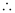Number of electrons that weigh 9.10939 × 10–31 kg = 1

Number of electrons that will weigh 1 g = (1 × 10–3 kg)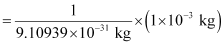= 0.1098 × 10–3 + 31

= 0.1098 × 1028

= 1.098 × 1027
(ii) Mass of one electron = 9.10939 × 10–31 kg

Mass of one mole of electron = (6.022 × 1023) × (9.10939 ×10–31 kg)

= 5.48 × 10–7 kg

Charge on one electron = 1.6022 × 10–19 coulomb

Charge on one mole of electron = (1.6022 × 10–19 C) (6.022 × 1023)

= 9.65 × 104 C

Q. 2.2
(i) Calculate the total number of electrons present in one mole of methane.
(ii) Find (a) the total number and (b) the total mass of neutrons in 7 mg of 14C. (Assume that mass of a neutron = 1.675 × 10–27 kg).
(iii) Find (a) the total number and (b) the total mass of protons in 34 mg of NH3 at STP.
Will the answer change if the temperature and pressure are changed?

Ans.
(i) Number of electrons present in 1 molecule of methane (CH4)

{1(6) + 4(1)} = 10

Number of electrons present in 1 mole i.e., 6.023 × 1023 molecules of methane

= 6.022 × 1023 × 10 = 6.022 × 1024
(ii)
(a) Number of atoms of 14C in 1 mole= 6.023 × 1023

Since 1 atom of 14C contains (14 – 6) i.e., 8 neutrons, the number of neutrons in 14 g of 14C is (6.023 × 1023) ×8.
Or, 14 g of 14C contains (6.022 × 1023 × 8) neutrons.

Number of neutrons in 7 mg: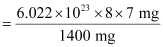= 2.4092 × 1021
(b) Mass of one neutron = 1.67493 × 10–27 kg

Mass of total neutrons in 7 g of 14C

= (2.4092 × 1021) (1.67493 × 10–27 kg)

= 4.0352 × 10–6 kg
(iii)
(a) 1 mole of NH3 = {1(14) + 3(1)} g of NH3

= 17 g of NH3

= 6.022× 1023 molecules of NH3

Total number of protons present in 1 molecule of NH3

= {1(7) + 3(1)}

= 10

Number of protons in 6.023 × 1023 molecules of NH3

= (6.023 × 1023) (10)

= 6.023 × 1024

⇒ 17 g of NH3 contains (6.023 × 1024) protons.

Number of protons in 34 mg of NH3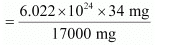= 1.2046 × 1022
(b) Mass of one proton = 1.67493 × 10–27 kg

Total mass of protons in 34 mg of NH3

= (1.67493 × 10–27 kg) (1.2046 × 1022)

= 2.0176 × 10–5 kg

The number of protons, electrons, and neutrons in an atom is independent of temperature and pressure conditions. Hence, the obtained values will remain unchanged if the temperature and pressure is changed.

Q. 2.3 How many neutrons and protons are there in the following nuclei?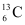,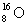,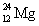,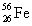,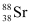Ans.
1. 136C

Atomic mass = 13

Atomic number = Number of protons = 6

Number of neutrons = (Atomic mass) – (Atomic number)

= 13 – 6 = 7

2.Atomic mass = 16

Atomic number = 8

Number of protons = 8

Number of neutrons = (Atomic mass) – (Atomic number)

= 16 – 8 = 8

3.Atomic mass = 24

Atomic number = Number of protons = 12

Number of neutrons = (Atomic mass) – (Atomic number)

= 24 – 12 = 12

4.Atomic mass = 56

Atomic number = Number of protons = 26

Number of neutrons = (Atomic mass) – (Atomic number)

= 56 – 26 = 30

5.Atomic mass = 88

Atomic number = Number of protons = 38

Number of neutrons = (Atomic mass) – (Atomic number)

= 88 – 38 = 50

Q. 2.4 Write the complete symbol for the atom with the given atomic number(Z) & Atomic mass(A).

(i) Z = 17, A = 35
(ii) Z = 92, A = 233
(iii) Z = 4, A = 9

Ans.

(i)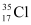(ii)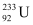(iii)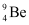Q. 2.5 Yellow light emitted from a sodium lamp has a wavelength (λ) of 580 nm. Calculate the frequency (ν) and wave number (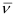) of the yellow light.

Ans. From the expression,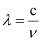We get,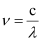…….. (i)

Where,

ν = frequency of yellow light

c = velocity of light in vacuum = 3 × 108 m/s

λ = wavelength of yellow light = 580 nm = 580 × 10–9 m

Substituting the values in expression (i):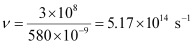Thus, frequency of yellow light emitted from the sodium lamp = 5.17 × 1014 s–1

Wave number of yellow light,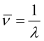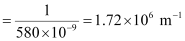Q. 2.6 Find energy of each of the photons which
(i) correspond to light of frequency 3× 1015 Hz.
(ii) have wavelength of 0.50 Å.

Ans.
(i) Energy (E) of a photon is given by the expression, E =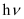Where, h = Planck’s constant = 6.626 × 10–34 Js

ν = frequency of light = 3 × 1015 Hz

Substituting the values in the given expression of E:

E = (6.626 × 10–34) (3 × 1015)

E = 1.988 × 10–18 J
(ii)  Energy (E) of a photon having wavelength (λ) is given by the expression,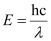h = Planck’s constant = 6.626 × 10–34 Js

c = velocity of light in vacuum = 3 × 108 m/s

Substituting the values in the given expression of E: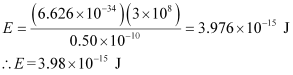Q. 2.7 Calculate the wavelength,frequency & wave number of a light wave whose period is 2.0×10–10s.
Ans. Frequency (ν) of light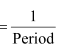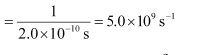Wavelength (λ) of light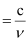Where, c = velocity of light in vacuum = 3×108 m/s

Substituting the value in the given expression of λ: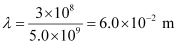Wave number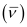of light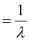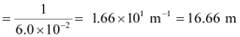Q. 2.8 What is the number of photons of light with a wavelength of 4000 pm that provide 1 J of energy?
Ans. Energy (E) of a photon = hν

Energy (En) of ‘n’ photons = nhν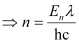Where,

λ = wavelength of light = 4000 pm = 4000 ×10–12 m

c = velocity of light in vacuum = 3 × 10m/s

h = Planck’s constant = 6.626 × 10–34 Js

Substituting the values in the given expression of n: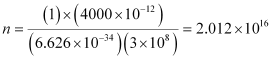Hence, the number of photons with a wavelength of 4000 pm and energy of 1 J are 2.012 × 1016.

Q. 2.9 A photon of wavelength 4 × 10–7 m strikes on metal surface, the work function of the metal being 2.13 eV. Calculate
(i) the energy of the photon (eV),
(ii) the kinetic energy of the emission, and
(iii) the velocity of the photo electron (1 eV= 1.6020 × 10–19 J).
Ans.
(i) Energy (E) of a photon = hν = hc/λ

Where,

h = Planck’s constant = 6.626 × 10–34 Js

c = velocity of light in vacuum = 3 × 10m/s

λ = wavelength of photon = 4 × 10–7 m

Substituting the values in the given expression of E: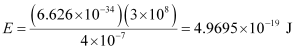Hence, the energy of the photon is 4.97 × 10–19 J.
(ii) The kinetic energy of emission Ek is given by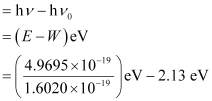= (3.1020 – 2.13) eV = 0.9720 eV

Hence, the kinetic energy of emission is 0.97 eV.
(iii) The velocity of a photo electron (ν) can be calculated by the expression,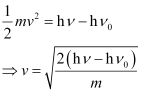Where,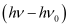is the kinetic energy of emission in Joules and ‘m’ is the mass of the photoelectron. Substituting the values in the given expression of v: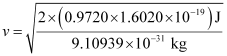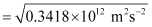v = 5.84 × 105 ms–1

Hence, the velocity of the photoelectron is 5.84 × 105 ms–1.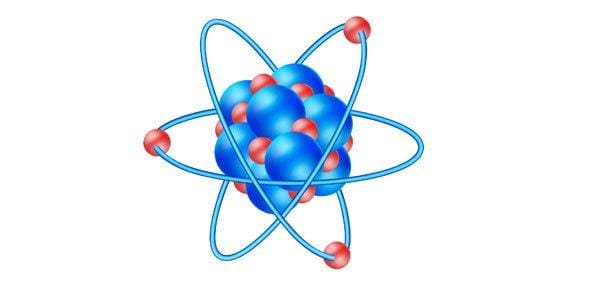AtomQ. 2.10 Electromagnetic radiation of wavelength 242 nm is just sufficient to ionise the sodium atom. Calculate the ionisation energy of sodium in kJ mol–1.
Ans. Energy of sodium (E)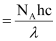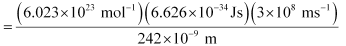= 4.947 × 105 J mol–1

= 494.7 × 103 J mol–1 = 494 kJ mol–1

Q. 2.11 A 25 watt bulb emits monochromatic yellow light of wavelength of 0.57μm. Calculate the rate of emission of quanta per second.
Ans. Power of bulb, P = 25 Watt = 25 Js–1

Energy of one photon, E = hν = hc/λ

Substituting the values in the given expression of E: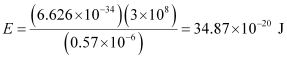E = 34.87 × 10–20 J

Rate of emission of quanta per second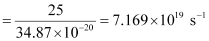Q. 2.12 Electrons are emitted with zero velocity from a metal surface when it is exposed to radiation of wavelength 6800 Å. Calculate threshold frequency (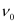) and work function (W0) of the metal.
Ans. Threshold wavelength of radian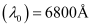= 6800 × 10–10 m

Threshold frequency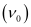of the metal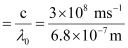= 4.41 × 1014 s–1

Thus, the threshold frequencyof the metal is 4.41 × 1014 s–1.

Hence, work function (W0) of the metal = hν0

= (6.626 × 10–34 Js) (4.41 × 1014 s–1)

= 2.922 × 10–19 J

Q. 2.13 What is the wavelength of light emitted when the electron in a hydrogen atom undergoes transition from an energy level with = 4 to an energy level with = 2?
Ans. The ni = 4 to nf = 2 transition will give rise to a spectral line of the Balmer series. The energy involved in the transition is given by the relation,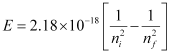Substituting the values in the given expression of E: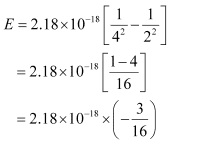E = – (4.0875 × 10–19 J)

Wavelength of light emitted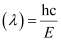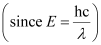Substituting the values in the given expression of λ: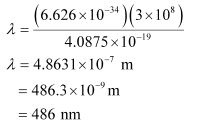Q. 2.14 How much energy is required to ionise a H atom if the electron occupies n = 5 orbit? Compare your answer with the ionization enthalpy of H atom (energy required to remove the electron from n = 1 orbit).
Ans. The expression of energy is given by,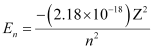Where,

Z = atomic number of the atom

n = principal quantum number

For ionization from n1 = 5 to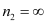,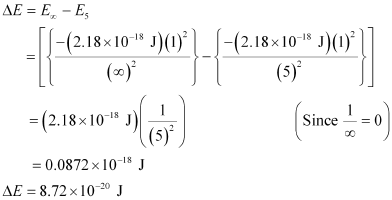Hence, the energy required for ionization from n = 5 to n =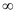is 8.72 × 10–20 J.

Energy required for n1 = 1 to n =,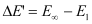Hence, less energy is required to ionize an electron in the 5th orbital of hydrogen atom as compared to that in the ground state.

Q. 2.15 What is the maximum number of emission lines when the excited electron of an H atom in n = 6 drops to the ground state?
Ans. When the excited electron of an H atom in n = 6 drops to the ground state, the following transitions are possible: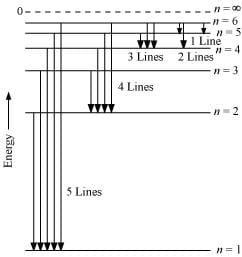Hence, a total number of (5 4 3 2 1) 15 lines will be obtained in the emission spectrum.

The number of spectral lines produced when an electron in the nth level drops down to the ground state is given by n(n-1)/2

Given, n = 6

Number of spectral lines =  6(6-1)/2 = 15.

Q. 2.16
(i) The energy associated with the first orbit in the hydrogen atom is –2.18 × 10–18 J atom–1. What is the energy associated with the fifth orbit?
(ii) Calculate the radius of Bohr’s fifth orbit for hydrogen atom.
Ans.
(i) Energy associated with the fifth orbit of hydrogen atom is calculated as: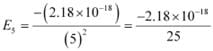E5 = –8.72 × 10–20 J
(ii) Radius of Bohr’s nth orbit for hydrogen atom is given by,

rn = (0.0529 nm) n2

For, n = 5

r5 = (0.0529 nm) (5)2

r5 = 1.3225 nm

Q. 2.17 Calculate the wave number for the longest wavelength transition in the Balmer series of atomic hydrogen.
Ans. For the Balmer series, ni = 2. Thus, the expression of wave number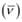is given by,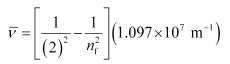Wave numberis inversely proportional to wavelength of transition. Hence, for the longest wavelength transition,has to be the smallest.

Forto be minimum, nf should be minimum. For the Balmer series, a transition from ni = 2 to nf = 3 is allowed. Hence, taking nf = 3, we get: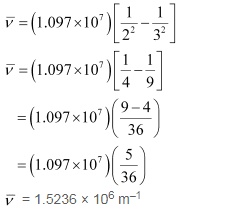Q. 2.18 What is the energy in joules, required to shift the electron of the hydrogen atom from the first Bohr orbit to the fifth Bohr orbit and what is the wavelength of the light emitted when the electron returns to the ground state? The ground state electron energy is –2.18 × 10–11 ergs.
Ans. Energy (E) of the nth Bohr orbit of an atom is given by,Where,

Z = atomic number of the atom

Ground state energy = – 2.18 × 10–11 ergs

= –2.18 × 10–11 × 10–7 J

= – 2.18 × 10–18 J

Energy required to shift the electron from n = 1 to n = 5 is given as: Δ= E5E1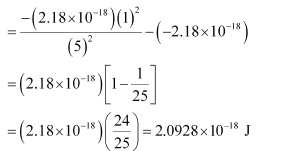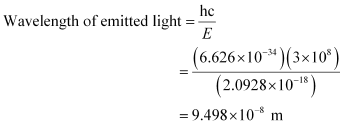Q. 2.19 The electron energy in hydrogen atom is given by En = (–2.18 × 10–18)/n2 J. Calculate the energy required to remove an electron completely from the n = 2 orbit. What is the longest wavelength of light in cm that can be used to cause this transition?
Ans. Given,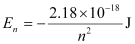Energy required for ionization from n = 2 is given by,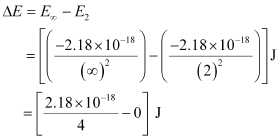ΔE = 0.545 × 10–18 J

= 5.45 × 10–19 J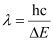Here, λ is the longest wavelength causing the transition.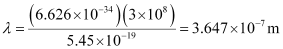= 3647 × 10–10 m

= 3647 Å

Q. 2.20 Calculate the wavelength of an electron moving with a velocity of 2.05 × 107 ms–1.
Ans. According to de Broglie’s equation,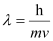Where,

λ = wavelength of moving particle

= mass of particle

v = velocity of particle

h = Planck’s constant

Substituting the values in the expression of λ: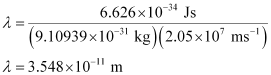Hence, the wavelength of the electron moving with a velocity of 2.05 × 107 ms–1 is 3.548 × 10–11 m.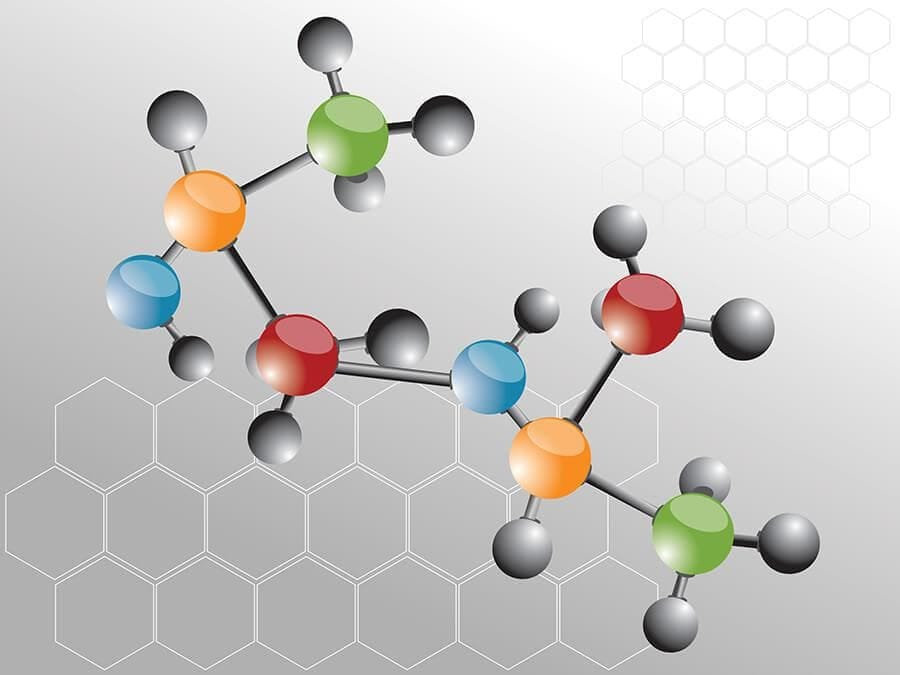Q. 2.21 The mass of an electron is 9.1 × 10–31 kg. If its K.E. is 3.0 × 10–25 J, calculate its wavelength.
Ans. From De Broglie’s equation,

λ = h/mv

Given,

Kinetic energy (K.E) of the electron = 3.0 × 10–25 J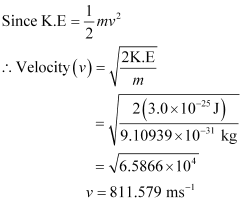Substituting the value in the expression of λ: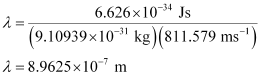Hence, the wavelength of the electron is 8.9625 × 10–7 m.

Q. 2.22 Which of the following are isoelectronic species i.e., those having the same number of electrons?

Na+ , K+ , Mg2+ , Ca2+ , S2–, Ar
Ans. Isoelectronic species have the same number of electrons.

Number of electrons in sodium (Na+) = 11

Number of electrons in (Na+ ) = 10

A positive charge denotes the loss of an electron.

Similarly,

Number of electrons in K+  = 18

Number of electrons in Mg2+  = 10

Number of electrons in Ca2+  = 18

A negative charge denotes the gain of an electron by a species.

Number of electrons in sulphur (S) = 16

∴ Number of electrons in S2- = 18

Number of electrons in argon (Ar) = 18

Hence, the following are isoelectronic species:

1) Na+ and Mg2+  (10 electrons each)

2) K+ , Ca2+ , S2– and Ar (18 electrons each)

Q. 2.23
(i) Write the electronic configurations of the following ions:

(a) H–
(b) Na+
(c) O2–
(d) F
(ii) What are the atomic numbers of elements whose outermost electrons are represented by:
(a) 3s1
(b) 2p3
(c) 3p5?
(iii) Which atoms are indicated by the following configurations?
(a) [He] 2s1
(b) [Ne] 3s2 3p3
(c) [Ar] 4s2 3d1.
Ans.
(i) (a) H ion

The electronic configuration of H atom is 1s1.

A negative charge on the species indicates the gain of an electron by it.

∴ Electronic configuration of H = 1s2

(b) Na+  ion

The electronic configuration of Na atom is 1s2 2s2 2p6 3s1.

A positive charge on the species indicates the loss of an electron by it.

∴ Electronic configuration of Na  = 1s2 2s2 2p6 3s0 or 1s2 2s2 2p6

(c) O2– ion

The electronic configuration of 0 atom is 1s2 2s2 2p4.

A dinegative charge on the species indicates that two electrons are gained by it.

∴ Electronic configuration of O2– ion = 1s2 2s2 p6

(d) F ion

The electronic configuration of F atom is 1s2 2s2 2p5.

A negative charge on the species indicates the gain of an electron by it.

∴ Electron configuration of F ion = 1s2 2s2 2p6

(ii) (a) 3s1

Completing the electron configuration of the element as

1s2 2s2 2p6 3s1.

∴ Number of electrons present in the atom of the element

= 2 + 2 + 6 + 1 = 11

∴ Atomic number of the element = 11

(b) 2p3

Completing the electron configuration of the element as

1s2 2s2 2p3.

∴ Number of electrons present in the atom of the element = 2 + 2 + 3 = 7

∴ Atomic number of the element = 7

(c) 3p5

Completing the electron configuration of the element as

1s2 2s2 2p5.

∴ Number of electrons present in the atom of the element = 2 + 2 + 5 = 9

∴ Atomic number of the element = 9

(iii) (a) [He] 2s1

The electronic configuration of the element is [He] 2s1 = 1s2 2s1.

∴ Atomic number of the element = 3

Hence, the element with the electronic configuration [He] 2s1 is lithium (Li).

(b) [Ne] 3s2 3p3

The electronic configuration of the element is [Ne] 3s2 3p3= 1s2 2s2 2p6 3s2 3p3.

∴ Atomic number of the element = 15

Hence, the element with the electronic configuration [Ne] 3s2 3pis phosphorus (P).

(c) [Ar] 4s2 3d1

The electronic configuration of the element is [Ar] 4s2 3d1= 1s2 2s2 2p6 3s2 3p6 4s2 3d1.

∴ Atomic number of the element = 21

Hence, the element with the electronic configuration [Ar] 4s2 3dis scandium (Sc).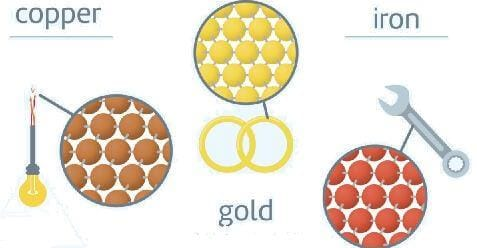Q. 2.24 What is the lowest value of n that allows g orbitals to exist?
Ans. For g-orbitals, = 4.

As for any value ‘n’ of principal quantum number, the Azimuthal quantum number (l) can have a value from zero to (n – 1).

∴ For l = 4, minimum value of n = 5

Q. 2.25 An electron is in one of the 3d orbitals. Give the possible values of n, l and ml for this electron.
Ans. For the 3d orbital:

Principal quantum number (n) = 3

Azimuthal quantum number (l) = 2

Magnetic quantum number (ml) = – 2, – 1, 0, 1, 2

The document NCERT Solutions: Structure of Atom - 1 - Notes | Study Chemistry Class 11 - NEET is a part of the NEET Course Chemistry Class 11.
All you need of NEET at this link: NEET

## Chemistry Class 11

204 videos|331 docs|229 tests
 Use Code STAYHOME200 and get INR 200 additional OFF

## Chemistry Class 11

204 videos|331 docs|229 tests

### How to Prepare for NEET

Read our guide to prepare for NEET which is created by Toppers & the best Teachers

Track your progress, build streaks, highlight & save important lessons and more!

,

,

,

,

,

,

,

,

,

,

,

,

,

,

,

,

,

,

,

,

,

;